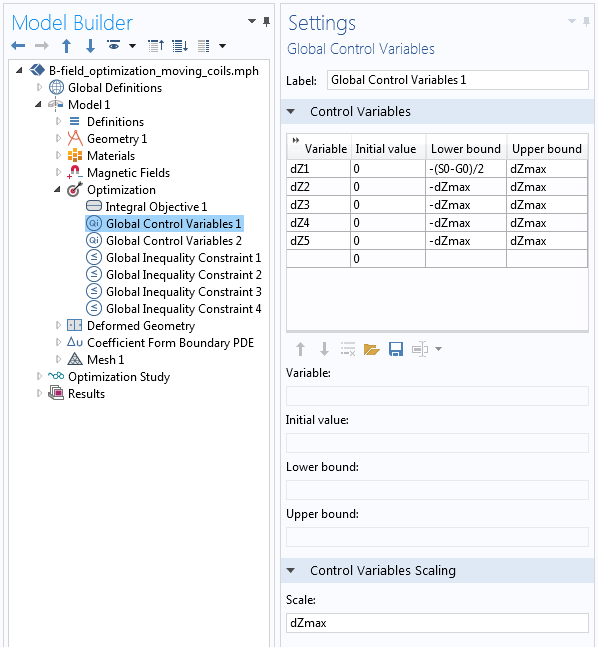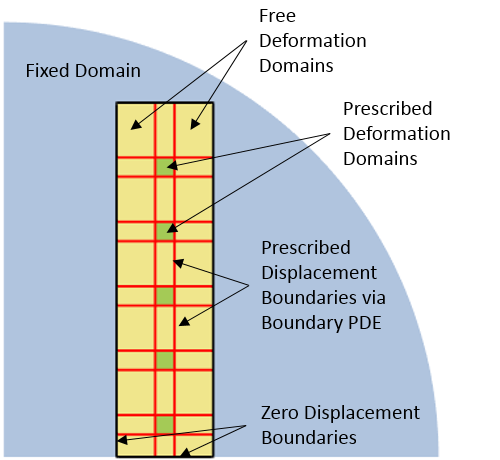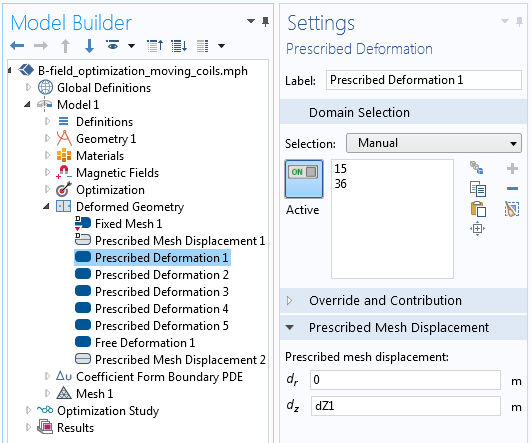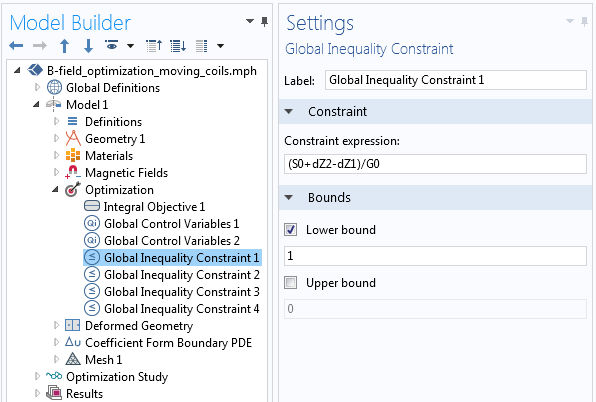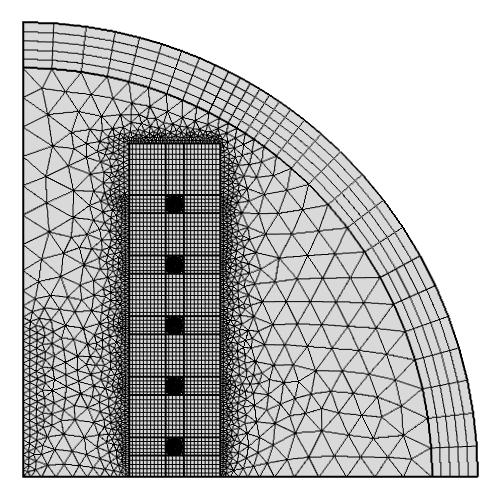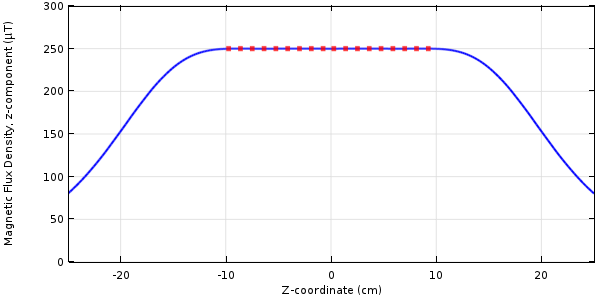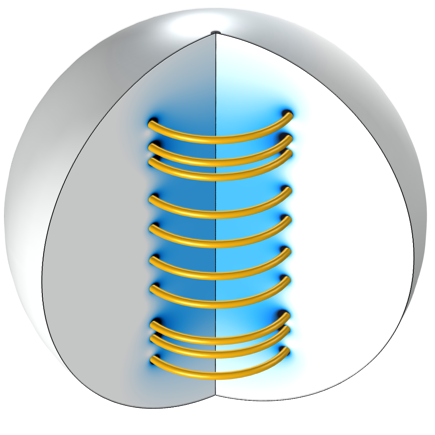# 如何优化电磁线圈的间距

2017年 4月 20日

### 初始线圈设计和优化问题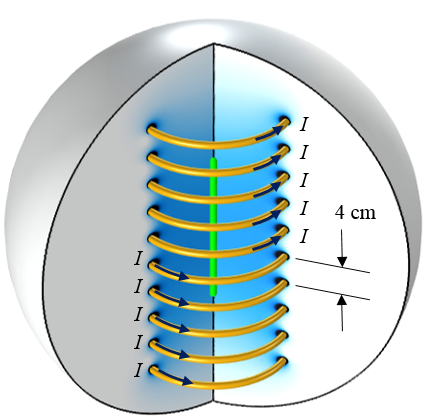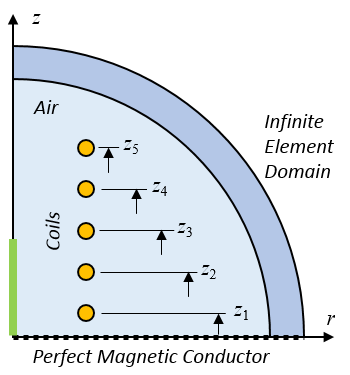\begin{aligned}& \underset{I, \Delta Z_1, \ldots ,\Delta Z_5}{\text{minimize:}}& & \frac{1}

{L_0}
\int_0^{L_0} \left( \frac{B_z}{B_0}
-1 \right) ^2 d l \\\end{aligned}

\begin{aligned}
& \text{subject to:}& & -(S_0-G_0)/2 \le \Delta Z_1 \leq \Delta Z_{max} \\\end{aligned}
\begin{aligned}
& & & -\Delta Z_{max} \leq \Delta Z_2, \ldots ,\Delta Z_5 \leq \Delta Z_{max} \\\end{aligned}
\begin{aligned}
& & & G_0 \le (Z_5-Z_4) \\\end{aligned}
\begin{aligned}
& & & G_0 \le (Z_4-Z_3) \\\end{aligned}
\begin{aligned}
& & & G_0 \le (Z_3-Z_2) \\\end{aligned}
\begin{aligned}
& & & G_0 \le (Z_2-Z_1) \\\end{aligned}
\begin{aligned}
& & & 0 \leq I \leq I_{max}\\\end{aligned}

### COMSOL Multiphysics® 中优化问题的建立和求解